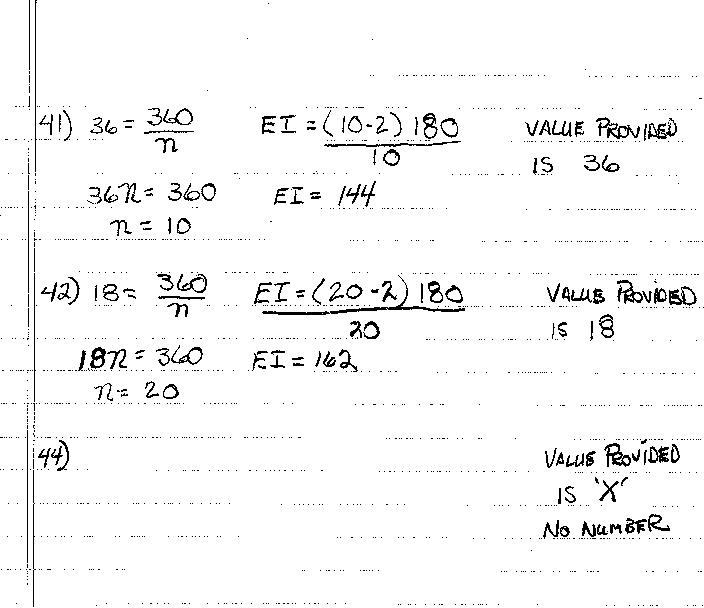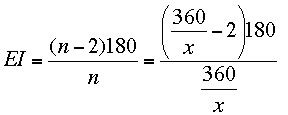SEARCH HOMEMath Central Quandaries & QueriesI'm a parent seeking help for my daughter. She seems to have most of this down but I have no clue. As you can see from the other problems she solved she can work the problem if a number is given. The 'X' value has us all lost. Here is the question and info she has. The question: The measure of an exterior angle of a regular polygon is given. Find the measure of an interior angle, and find the sides. 41. 36 42. 18 44. 'X' The attachment has what she has done for 41 and 42. Need help with 44 (lots of help) Thank you in advance for your site and your help. RobertHi Robert,

From what you sent your daughter does know what she is doing.She is already working with a variable, n. For example in 41) she starts with 36 = 360/n, multiplies both sides by n to get 36n = 360 and then divides both sides by 36 to obtain n = 10. Then she substitutes n = 10 into the expression for EI to find the measure of the interior angle.

For 44) she needs to perform the same steps even though she doesn't know the value of x. The first expression is then

x = 360/n

Now, as she did before multiply both sides by n to obtain

n × x = 360.

Her next step was to divide both sides by the value of x and this gives

n = 360/x.

This just says that the number of sides is 360 divided by the measure of the exterior angle.

Then substitute this value for n into the expression for EII think I will leave it at this point. This does simplify to become a much nicer expression but I expect that is what they will do next in class.

I hope this helps,
PennyMath Central is supported by the University of Regina and The Pacific Institute for the Mathematical Sciences.